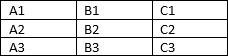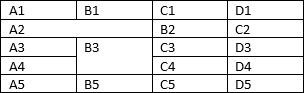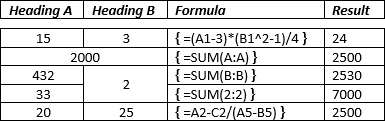# How to reference a cell of a Word table

Word
If you have a table in the Word document, you can insert some formulas and functions in it without using an Excel table. It is easy and fast to insert a formula to the Word table that use the values of some table cells.

In the formula you can use a cell address to refer to a cell. A cell address format for the Word table is the same as in Excel:

• Columns are named as A, B, C, etc.
• Rows are named as 1, 2, 3, etc.:See How to add, subtract, multiply and divide cells in a Word table for more details.

So, when you create a formula, you can use a cell address as a name of column plus name of its row. For example:

• a cell in the first column and the second row has an address A2,
• a cell in the third column and the third row has an address C3.

Attention!

1. Be very careful, if you have merged cells:
• The merged cells of one row change the column numbers for other cells.
• The merged cells of one column don't change the row number for other cells:For example:In this example, there are two different tables to show formulas of calculating sum of entire column, the value of cell B4 is zero (0) because this cell is missed.

We strongly recommend avoiding merged cells!

2. Be very careful with values in the table. All formulas and functions work only with numbers. So, you can't use in cells that used in formulas any character except:
• Digits (0-9),
• Decimal symbol (can be a decimal point or a comma),
• Digit grouping symbol (can be a comma or a decimal point),
• Negative number symbol (usually -).

You can't use the currency symbols or separate numbers by spaces, etc.

See also this tip in French: Comment référencer une cellule d'un tableau Word.